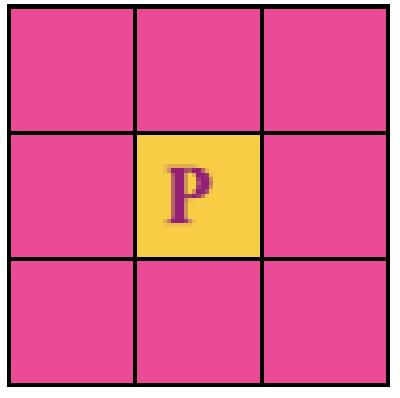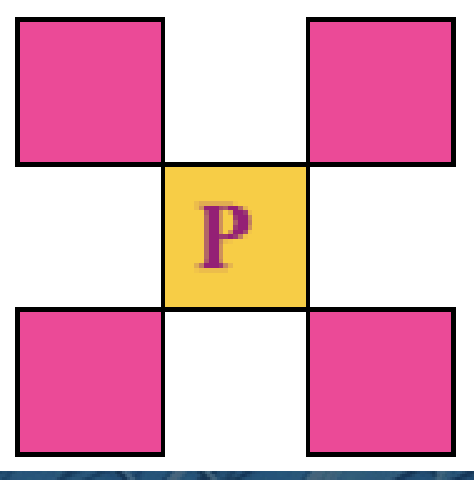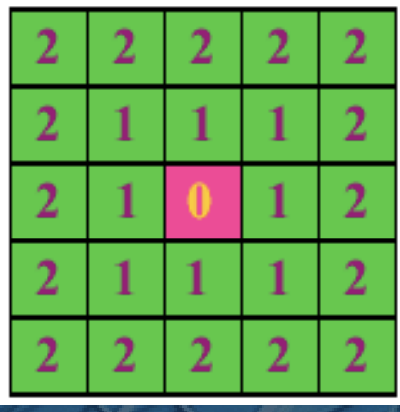## 邻域

• 4-邻域 ( $N_{4} (p)$ )• 8-邻域 ( $N_{8}(p)$ )• 对角邻域 ( $N_{D}(p)$ )## 邻接

• 4-邻接

• 如果q在N4(p)中，满足灰度值条件为集合V 的两个像素p和q是4邻接的。

• 例：V={1}，且p、q的灰度值都为1.

| 0 | 0 | 0 |
| 0 | p | q |
| 0 | 0 | 1 |

• 8-邻接

• 如果q在N8(p)中，满足灰度值条件为集合V 的两个像素p和q是8邻接的。

• 例：V={1}，且p、q的灰度值都为1.

| 0 | 1 | 0 |
| 0 | p | 0 |
| 0 | 0 | q |

• m-邻接

• 灰度值满足集合V的像素 p和q，若符合下列 两个条件之一：
• a、q在p的4邻域中；
• b、q在p的对角领域中，并且q的4邻域与p的 4领域交集像素中没有灰度值属于V。则称p , q两点是m邻接。

## 连通

$$[(x_{0},y_{0}),(x_{1},y_{1}), … ,(x_{n},y_{n}),]$$

$n$ 称为通路的长度,根据邻接类型，分为：

• 4-通路
• 8-通 路
• m-通路。

• 4-连通
• 8-连通
• m-连通。

## 距离

1. $D(p,q) \ge 0$ (当切仅当$p=q$时等号成立)
2. $D(p,q)=D(q,p)$
3. $D(p,r) \le D(p,q) + D(q,r)$

• 欧式距离

$$D_{e}(p,q)=[(x-s)^{2}+(y-t)^{2}]^{\frac{1}{2}}$$

距离p的欧式距离小于或等于某一值R的像素都包含在以 p 为圆心且半径为R的圆内。

• 城区距离

$$D_{4}(p,q)=|x-s|+|y-t|$$• 棋盘距离

$$D_{8}(p,q)=max(|x-s|,|y-t|)$$欧式距离给出的结果比较准确，但计算时要进行平方和开方运算，计算量大。 城区距离和棋盘距离均为非欧式距离， 计算量小，但有一定的误差